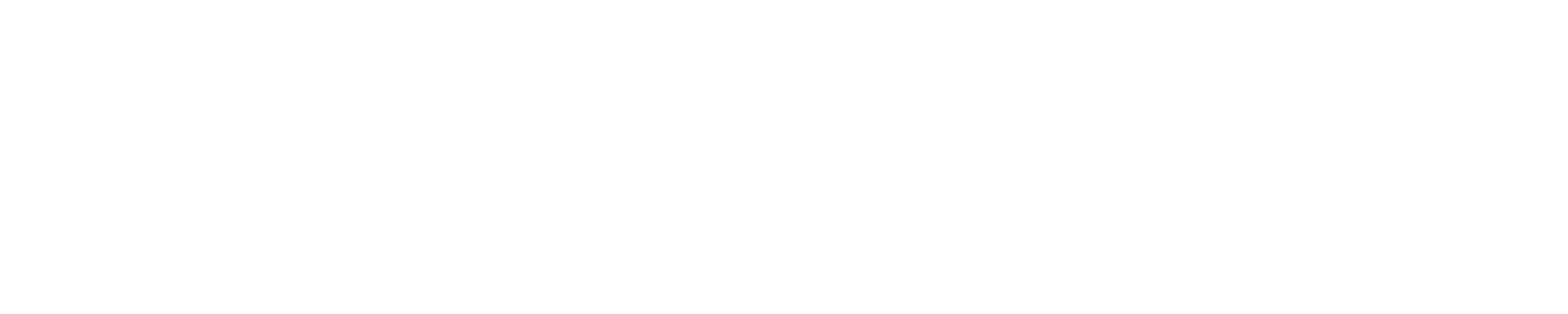# Help needed around importing historic statistics

Hello. I develop an integration for managing a users energy from the supplier Octopus Energy. Unfortunately, for most users the data is not available in real time and is usually available the next day. I have a sensor that represents the accumulative consumption for the previous day, which changes daily. This sensor has a device_class of `Energy`, state_class of `total` and unit_of_measurement of `kwh`. The last_reset is set to the start of the day that the accumulation represents.

I’m now trying to import this data in the 30 minute increments that are available so that it can appear nicely in the Energy Dashboard. As you can see below I’m successfully importing the data

However in doing so, I’m still having spikes for today that represents the accumulation which I’m trying to remove.

Is this even possible? Below you can see the logic for importing the statistics and was wondering if anyone has any advice if this is possible.

``````async def async_import_statistics_from_consumption(hass: HomeAssistant, now: datetime, unique_id: str, name: str, consumptions, unit_of_measurement: str, consumption_key: str):
statistic_id = f"sensor.{unique_id}".lower()

# Our sum needs to be based from the last total, so we need to grab the last record from the previous day
statistics_during_period,
hass,
consumptions["from"] - timedelta(days=7),
consumptions["from"],
{statistic_id},
"hour",
None,
{"sum"}
)

statistics = []

last_reset = consumptions["from"].replace(minute=0, second=0, microsecond=0)
sum = last_stat[statistic_id][-1]["sum"] if statistic_id in last_stat and len(last_stat[statistic_id]) > 0 else 0
state = 0

_LOGGER.debug(f'last_stat: {last_stat}; sum: {sum}; last_reset: {last_reset}')

for index in range(len(consumptions)):
charge = consumptions[index]

start = charge["from"].replace(minute=0, second=0, microsecond=0)
sum += charge[consumption_key]
state += charge[consumption_key]

_LOGGER.debug(f'index: {index}; start: {start}; sum: {sum}; state: {state}; added: {(index) % 2 == 1}')

if index % 2 == 1:
statistics.append(
StatisticData(
start=start,
last_reset=last_reset,
sum=sum,
state=state
)
)

# # Fill up to now to wipe the capturing of the data on the entity
target = now - timedelta(hours=1)
current = start + timedelta(hours=1)
while (current < target):
statistics.append(
StatisticData(
start=current,
last_reset=last_reset,
sum=sum,
state=state
)
)

_LOGGER.debug(f'start: {current}; sum: {sum}; state: {0};')

current = current + timedelta(hours=1)

has_mean=False,
has_sum=True,
name=name,
source='recorder',
statistic_id=statistic_id,
unit_of_measurement=unit_of_measurement,
)

``````

hi dave,
super interested in the outcome of this, im really struggling to get it working i can only manage the “accumulation” once a day around midday.I am in no way a dev, I’m a HA n00b, i dont really know yaml… so not really of much help from an experience point of view.
having said that a certain AI Platform suggests, removing

``````    statistics_during_period,
hass,
consumptions["from"] - timedelta(days=7),
consumptions["from"],
{statistic_id},
"hour",
None,
{"sum"}
)

sum = last_stat[statistic_id][-1]["sum"] if statistic_id in last_stat and len(last_stat[statistic_id]) > 0 else 0
``````

and inserting

``````
for index in range(len(consumptions)):
charge = consumptions[index]

start = charge["from"].replace(minute=0, second=0, microsecond=0)
sum += charge[consumption_key]
state += charge[consumption_key]
``````

im not sure how much your code relies on the “last total” also in my experience, AI gets it wrong on first few attempts.

im not sure on where to put the code, else i would have tested before posting up. apologies if this is just noise

I’m afraid AI has failed youYou need to have previous sums otherwise you end up with even weirder results. I think the issue is the difference between long and short term statistics in HA. However there’s no way of injecting short term statistics so this might just be the best I can do.

I currently have a solution for this problem by importing and exposing external statistics, but it’s just not as intuitve to setup.

…againI didnt think I’d be able to offer anything useful. The “solution” sounds promising though. When/if youre ready to share with the community I’d love to have a stab it.

i have other issues at the moment, need to sort those before tweaking

From your other post, the external statistics approach has already been released

As per the latest release and instructions you need to use the external statistics (which can take up to 24 hours to appear) instead of the entities in order to get more granular data in the energy dashboard. The dashboard will never look identical to the apps as the HA dashboard only displays data in hourly chunks (not half hourly).

1 Like